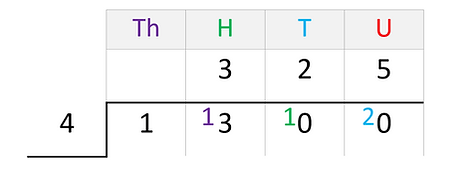top of page

## Topic 4: Calculating with integersThe following questions are typical at Level 1. The written methods shown here are recommended, but you may know and use other methods. What is important is that you always show all of your working out.

Example: 838 + 714

Method:

Arrange the two numbers in columns.

Find the total of the units column.    8 + 4 = 12, which is  1  ten and  units. Regroup the ten into the tens column.

Find the total of the tens column. 3 tens  +1 ten + 1 ten =  tens.

Find the total of the hundreds column. 8 hundreds + 7 hundreds = 15 hundreds, which is  1  thousand and  hundreds. Regroup the 1 thousand into the thousands column.

838 + 714 = 1552

Example: 695 + 881 + 62

Method:

Arrange the three numbers in columns.

Find the total of the units column. 5 + 1 + 2 =  8 .

Find the total of the tens column. 9 tens + 8 tens + 6 tens = 23 tens, which is  hundreds and  tens. Regroup the 2 hundreds into the hundreds column.

Find the total of the hundreds column. 6 hundreds + 8 hundreds + 2 hundreds = 16 hundreds, which is  thousand and  6  hundreds. Regroup the 1 thousand into the thousands column.

695 + 881 + 62 = 1638

Subtraction with regrouping

Example: 432 – 268

Method:

Arrange the two numbers in columns.

Complete the units column first. You can't take 8 from 2. Regroup   ten into the units column, leaving  2  tens in the tens column.

Now the units column can be completed. 12 8 = 4.

Complete the tens column next. You can't take 6 tens from 2 tens. Regroup  1  hundred into the tens column, leaving  3  hundreds in the hundreds column.

Now the tens column can be completed. 12 tens 6 tens = 6 tens.

Finally, complete the hundreds column. 3 hundreds – 2 hundreds = 1 hundred.

432 – 268 = 164

Subtraction with the 'zero problem' (or the 'empty column problem')

Example: 704 – 459

Method:

Arrange the two numbers in columns.

Complete the units column first. You can't take 9 from 4 so you need to regroup a ten into the units column. However, the tens column is empty.

Regroup  1  hundred into the tens column, leaving  6  hundreds in the hundreds column. Now you can regroup   ten into the units column, leaving  9  tens in the tens column.

Now the units column can be completed. 14  9 = 5.

Next the tens column can be completed. 9 tens  5 tens = 4 tens.

Finally the hundreds column can be completed. 6 hundreds  4 hundreds = 2 hundreds.

704 – 459 = 245

Multiplication with regrouping

Example: 187 × 5

Method:

Arrange the number to be multiplied in columns, with an answer box below, as you would for an addition.

Complete the units column first. × 5 = 35, which is  3  tens and  5  units. Regroup the 3 tens into the tens column.

Next complete the tens column. 8 tens × 5 = 40 tens. Add the 5 regrouped tens to make 45 tens, which is  4  hundreds and  tens. Regroup the 4 hundreds into the hundreds column.

Finally complete the hundreds column. 1 hundred × 5 = 5 hundreds. Add the 4 regrouped hundreds to make  hundreds.

187 × 5 = 985

Division with regrouping

Example: 1 300 ÷ 4

Method:

Most people regroup for division differently than for the other operations, by beginning with the furthest column to the left. The calculation is usually laid out differently too (see below).

In the thousands column, 4 thousands will not go into 1 thousand, so the  1  thousand is regrouped into the hundreds column.

In the hundreds column, 4 hundreds will go into 13 hundreds 3 times, with  hundred remaining. This hundred is regrouped into the tens column.

In the tens column, 4 tens will go into 10 tens 2 times,

with  tens remaining. These tens are regrouped into the units column.

Finally, in the units column, 4 will go into 20 5 times.

1 300 ÷ 4 = 325bottom of page